Select Region
Worldwide

# Calculation Bases

## Design of Industrial Shock Absorbers

ACE shock absorbers provide linear deceleration and are therefore superior to other kinds of damping element. With the help of the overview of the calculation bases for the design of a shock absorber you will be able to select the right industrial shock absorber for your project from the ACE web catalogue.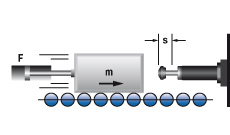It is easy to calculate around 90 % of applications knowing only the following five parameters:

1. Mass to be decelerated (weight)                     m    [kg]
2. Impact velocity at shock absorber                 vD   [m/s]
3. Propelling force                                                F     [N]
4. Cycles per hour                                                c      [1/h]
5. Number of absorbers in parallel                     n

## Key to symbols used

SymbolUnitDescriptionSymbolUnitDescription
WNmKinetic energy per cycle3ST1 to 3tall torque factor (normally 2.5)
WNmPropelling force energy per cycleMNmPropelling torque
W3NmTotal energy per cycle (W1 + W2)Ikgm2Moment of Inertia
1W4Nm/hrTotal energy per hour (W3 · c)gm/s2Acceleration due to gravity = 9.81
mekgEffective weighthmDrop height excl. shock absorber stroke
mkgMass to be deceleratedsmShock absorber stroke
nNumber of shock absorbers (in parallel)L/R/rmRadius
2vm/sVelocity at impactQNReaction force
2vDm/sImpact velocity at shock absorberμCoefficient of friction
FNPropelling forceam/s2Deceleration
PkWMotor powerβ°Angle of incline
1 All mentioned values of W4 in the capacity charts are only valid for room temperature. There are reduced values at higher temperature ranges.
2 v or vD is the final impact velocity of the mass. With accelerating motion the final impact velocity can be 1.5 to 2 times higher than the average. Please take this into account when calculating kinetic energy.
3 ST =^ relation between starting torque and running torque of the motor (depending on the design)
In all the following examples the choice of shock absorbers made from the capacity chart is based upon the values of (W3), (W4), (me) and the desired shock absorber stroke (s).

Note:
When using several shock absorbers in parallel, the values (W3), (W4) and (me) are divided according to the number of units used.

 Reaction force Q [N]Q = (1.5 · W3) / s Stopping time t [s]t = (2.6 · s) / vD Deceleration a [m/s2]a = (0.75 · vD2) / s
Approximate values assuming correct adjustment. Add safety margin if necessary. (Exact values will depend upon actual application data and can be provided on request.)

## Applications

ApplicationFormulaeExample

### 1. Mass without propelling force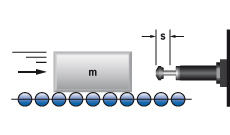W1 = m · v2 · 0.5
W2 = 0
W3 = W1 + W2
W4 = W3 · c
vD = v
me = m

m = 100 kg
v = 1.5 m/s
x = 500 1/hr
s = 0.050 m (chosen)

W1 = 100 · 1.52 · 0.5 = 113 Nm
W2 = 0
W3 = 113 + 0 = 113 Nm
W4 = 113 · 500 = 56500 Nm/hr
me = m = 100 kg

### 2. Mass with propelling forceW1 = m · v2 · 0.5
W2 = F · s
W3 = W1 + W2
W4 = W3 · c
vD = v
me = (2 · W3) / vD2
2.1 for vertical motion upwards
W2 = (F – m · g) · s
2.2 for vertical motion downwards
W2 = (F + m · g) · s
m = 36 kg
1v = 1.5 m/s
F = 400 N
c = 1000 1/hr
s = 0.025 m (chosen)

W1 = 36 · 1.52 · 0.5 = 41 Nm
W2 = 400 · 0.025 = 10 Nm
W3 = 41 + 10 = 51 Nm
W4 = 51 · 1000 = 51000 Nm/hr
me = 2 · 51 : 1.52 = 45 kg

1 v is the fi nal impact velocity of the mass:
With pneumaticallypropelled systems this can be 1.5 to 2 times the average velocity.
Please take this into account when calculating energy.

### 3. Mass with motor drive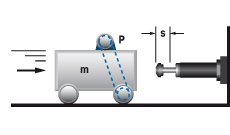W1 = m · v2 · 0.5
W2 = (1000 · P ·ST · s) / v
W3 = W1 + W2
W4 = W3 · c
vD = v
me = (2 · W3) / vD2

m = 800 kg
v = 1.2 m/s
ST = 2.5
P = 4 kW
x = 100 /hr
s = 0.100 m (chosen)

W1 = 800 · 1.22 · 0.5 = 576 Nm
W2 = 1000 · 4 · 2.5 · 0.1 : 1.2 = 834 Nm
W3 = 576 + 834 = 1410 Nm
W4 = 1410 · 100 = 141000 Nm/hr
me = 2 · 1410 : 1.22 = 1958 kg

Note: Do not forget to include the rotational energy of
motor, coupling and gearbox into calculation for W1.

### 4. Mass on driven rollers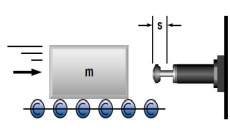W1 = m · v2 · 0.5
W2 = m · μ · g · s
W3 = W1 + W2
W4 = W3 · c
vD = v
me = (2 · W3) / vD2

m = 250 kg
v = 1.5 m/s
c = 180 1/h
(Steel/Steel) μ = 0.2
s = 0.050 m (chosen)

W1 = 250 · 1.52 · 0.5 = 281 Nm
W2 = 250 · 0.2 · 9.81 · 0.05 = 25 Nm
W3 = 281 + 25 = 306 Nm
W4 = 306 · 180 = 55080 Nm/hr
me = 2 · 306 : 1.52 = 272 kg

### 5. Swinging mass with propelling forceW1 = m · v2 · 0.5 = 0.5 · I · ω2
W2 = (M · s) / R
W3 = W1 + W2
W4 = W3 · c
vD = (v · R) / L = ω · R
me = (2 · W3) / vD2

m = 20 kg
v = 1 m/s
M = 50 Nm
R = 0.5 m
L = 0.8 m
c = 1500 1/h
s = 0.012 m (chosen)

W1 = 20 · 12 · 0.5 = 10 Nm
W2 = 50 · 0.012 : 0.5 = 1.2 Nm
W3 = 10 + 1.2 = 11.2 Nm
W4 = 306 · 180 = 16800 Nm/hr
vD  = 1 · 0.5 : 0.8 = 0.63 m/s
me = 2 · 11.2 : 0.632 = 56 kg

### 6. Free falling mass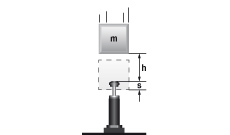W1 = m · g · h
W2 = m · g · s
W3 = W1 + W2
W4 = W3 · c
vD = √2 · g · h
me = (2 · W3) / vD2

m = 30 kg
h = 0.5 m
c = 400 /hr
s = 0.050 m (chosen)

W1 = 30 · 0.5 · 9.81 = 147 Nm
W2 = 30 · 9.81 · 0.05 = 15 Nm
W3 = 147 + 15 = 162 Nm
W4 = 162 · 400 = 64800 Nm/hr
vD  = √2 · 9.81 · 0.5 = 3.13 m/s
me = 2 · 162 : 3.132 = 33 kg

### 6.1 Mass rolling/sliding down inclineW1 = m · g · h = m · vD2 · 0.5
W2 = m · g · sinβ · s
W3 = W1 + W2
W4 = W3 · c
vD = √2 · g · h
me = (2 · W3) / vD2

6.1a propelling force up incline
W2 = (F – m · g· sinβ) · s
6.1b propelling force down incline
W2 = (F + m · g· sinβ) · s

m = 500 kg
h = 0.1 m
c = 200 /hr
ß = 10 °C

W1 = 500 · 9.81 · 0.1 = 490.5 Nm
W2 = 50 · 9.81 · sin(10) · 0.075 = 63.9 Nm
W3 = 490.5 + 63.9 = 554.4 Nm
W4 = 554.4 · 200 = 11880.0 Nm/hr

### 6.2 Mass free falling about a pivot point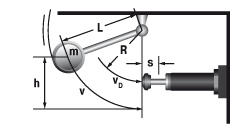W1 = m · g · h
W2 = 0
W3 = W1 + W2
W4 = W3 · c
vD = √2 · g · h · (R / L)
me = (2 · W3) / vD2

tan α = s / R

m = 50 kg
h = 1 m
c = 50 /hr
R = 300 mm
L = 500 mm

W1 = 50 · 9.81 · 1 = 490.5 Nm
W2 = 0
W3 = 490.5 + 0 = 490.5 Nm
W4 = 490.5 · 50 = 24525.0 Nm/hr

### 7. Rotary index table with propelling torque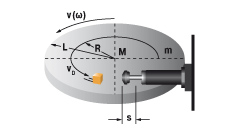W1 = m · v2 · 0.25 = 0.5 · I · ω2
W2 = (M · s) / R
W3 = W1 + W2
W4 = W3 · c
vD = (v · R) / L = ω · R
me = (2 · W3) / vD2

m = 1000 kg
v = 1.1 m/s
M = 1000 Nm
s = 0.050 m (chosen)
L = 1.25 m
R = 0.8 m
c = 100 /hr

W1 = 1000 · 1.12 · 0.25 = 303 Nm
W2 = 300 · 0.025 : 0.8 = 63 Nm
W3 = 28 + 9 = 366 Nm
W4 = 37 · 1200 = 36600 Nm/hr
vD = 1.1 · 0.8 : 1.25 = 0.7 m/s
me = 2 · 366 : 0.72 = 1494 kg

### 8. Swinging arm with propelling torque (uniform weight distribution)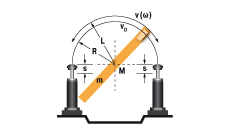W1 = m · v2 · 0.17 = 0,5 · I · ω2
W2 = (M · s) / R
W3 = W1 + W2
W4 = W3 · c
vD = (v · R) / L = ω · R
me = (2 · W3) / vD2

I = 56 kgm2
M = 300 Nm
s = 0,025 m (chosen)
L = 1.5 m
R = 0.8 m
c = 1200 /hr

W1 = 0.5 · 56 · 12 = 28 Nm
W2 = 300 · 0.025 : 0.8 = 9 Nm
W3 = 28 + 9 = 37 Nm
W4 = 37 · 1200 = 44400 Nm/hr
vD = 1 · 0.8 = 0.8 m/s
me = 2 · 37 : 0.82 = 116 kg

### 9. Swinging arm with propelling force (uniform weight distribution)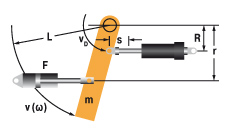W1 = m · v2 · 0.17 = 0.5 · I · ω2
W2 = (F · r · s) / R = (M · s) / R
W3 = W1 + W2
W4 = W3 · c
vD = (v · R) / L = ω · R
me = (2 · W3) / vD2

m = 1000 kg
v = 2 m/s
F = 7000 N
M = 4200 Nm
s = 0.050 m (chosen)
r = 0.6 m
R = 0.8 m
L = 1.2 m
c = 900 /hr

W1 = 1000 · 22 · 0.17 = 680 Nm
W2 = 7000 · 0.6 · 0.05 : 0.8 = 263 Nm
W3 = 680 + 263 = 943 Nm
W4 = 943 · 900 = 848700 Nm/hr
vD = 2 · 0.8 : 1.2 = 1.33 m/s
me = 2 · 943 : 1.332 = 1066 kg

### 10. Mass lowered at controlled speed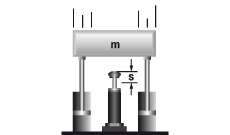W1 =m · v2 · 0.5
W2 = m · g · s
W3 = W1 + W2
W4 = W3 · c
vD = v
me = (2 · W3) / vD2

m = 6000 kg
v = 1.5 m/s
s = 0.305 m (chosen)
c = 60 /hr

W1 = 6000 · 1.52 · 0.5 = 6750 Nm
W2 = 6000 · 9.81 · 0.305 = 17952 Nm
W3 = 6750 + 17952 = 24702 Nm
W4 = 24702 · 60 = 1482120 Nm/hr
me = 2 · 24702 : 1.52 = 21957 kg

## Effective Weight (me)

The effective weight (me) can either be the same as the actual weight (examples Aand C), or it can be an imaginary weight representing a combination of the propelling force or lever action plus the actual weight (examples B and D).

EinsatzfallBeispiel

### A    Mass without propelling forcem = 100 kg
vD = v = 2 m/s
W1 = W3 = 200 Nm
me = (2 · 200) / 4 = 100 kg

Formula: me = m

### B    Mass with propelling forcem = 100 kg
F = 2000 N
vD = v = 2 m/s
s = 0.1 m
W1 = 200 Nm
W2 = 200 Nm
W3 = 400 Nm
me = (2 · 400) / 4 = 200 kg

Formula: me = (2 · W3) / vD2

### C    Mass without propelling force direct       against shock absorber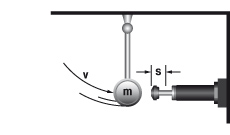m = 20 kg
vD = v = 2 m/s
W1 = W3 = 40 Nm
me = (2 · 40) / 22 = 20 kg

Formula: me = m

###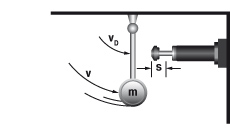m = 20 kg
v = 2 m/s
vD = 0.5 m/s
s = 0.1 m
W1 = W3 = 40 Nm
me = (2 · 40) / 0.52 = 320 kg

Formula: me = (2 · W3) / vD2
×